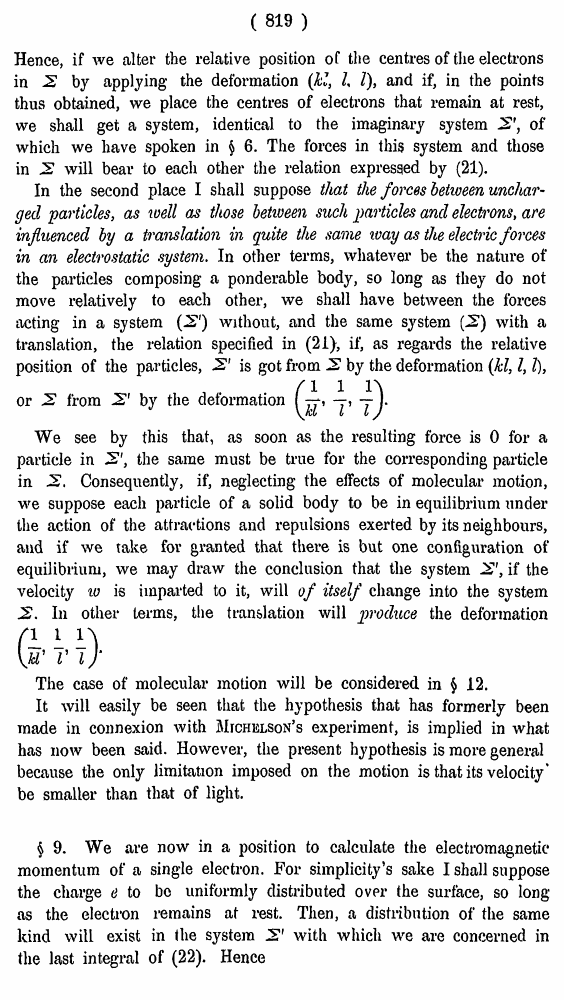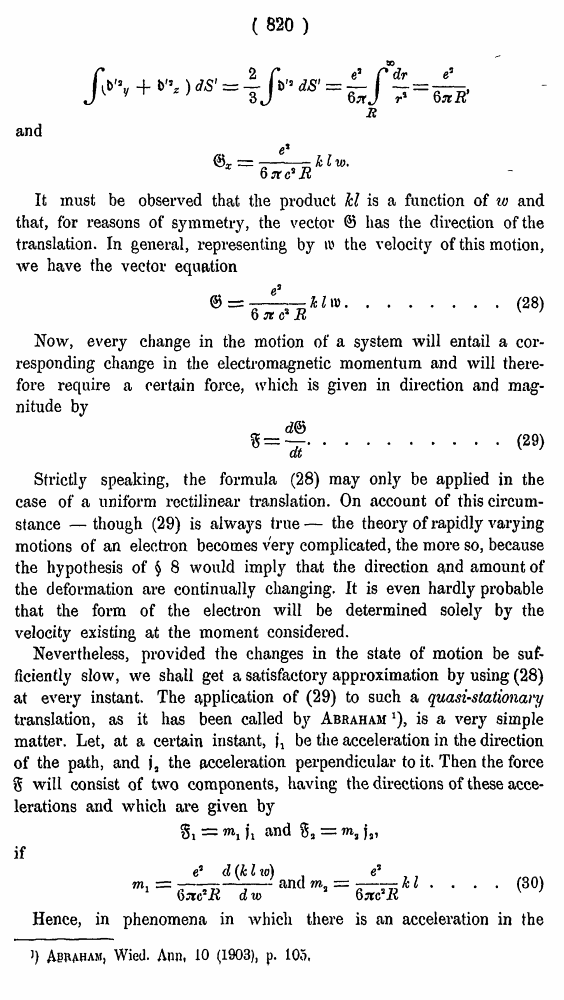# H. A. Lorentz. Electromagnetic phenomena in a system moving with any velocity smaller than that of light. // Proceedings Royal Acad., Amsterdam. Vol. VI., 1904

В начало   Другие форматы   <<<     Страница 819   >>>809  810  811  812  813  814  815  816  817  818  819 820  821  822  823  824  825  826  827  828  829  830  831 ( 819 ) Hence, if we alter the relative position of the centres of the electrons in 2 by applying the deformation (kl, I, I), and if, in the points thus obtained, we place the centres of electrons that remain at rest, we shall get a system, identical to the imaginary system 2', of which we have spoken in § 6. The forces in this system and those in 2 will bear to each other the relation expressed by (21). In the second place I shall suppose that the forces between uncharged particles, as well as those between such particles and electrons, are influenced by a translation in quite the same ivay as the electric forces in an electrostatic system. In other terms, whatever be the nature of the particles composing a ponderable body, so long as they do not move relatively to each other, we shall have between the forces acting in a system (2") without, and the same system (2) with a translation, the relation specified in (21), if, as regards the relative position of the particles, 2' is got from 2 by the deformation (kl, I, I), We see by this that, as soon as the resulting force is 0 for a particle in 2', the same must be true for the corresponding particle in 2, Consequently, if, neglecting the effects of molecular motion, we suppose each particle of a solid body to be in equilibrium under the action of the attractions and repulsions exerted by its neighbours, and if we take for granted that there is but one configuration of equilibrium, we may draw the conclusion that the system 2', if the velocity to is imparted to it, will of itself change into the system 2. In other terms, the translation will produce the deformation The case of molecular motion will be considered in § 12. It will easily be seen that the hypothesis that has formerly been made in connexion with Michelson's experiment, is implied in what has now been said. However, the present hypothesis is more general because the only limitation imposed on the motion is that its velocity* be smaller than that of light. \$ 9. We are now in a position to calculate the electromagnetic momentum of a single electron. For simplicity’s sake I shall suppose the charge e to be uniformly distributed over the surface, so long as the electron remains at rest. Then, a distribution of the same kind will exist in the system 2' with which we are concerned in the last integral of (22). Hence or 2 from 2' by the deformation ( 820 ) and It must be observed that the product hi is a function of w and that, for reasons of symmetry, the vector <3 has the direction of the translation. In general, representing by ti' the velocity of this motion, we have the vector equation .......(28) Now, every change in the motion of a system will entail a corresponding change in the electromagnetic momentum and will there-foi’e require a certain force, which is given in direction and magnitude by ........(29) Strictly speaking, the formula (28) may only be applied in the case of a uniform rectilinear translation. On account of this circumstance — though (29) is always true — the theory of rapidly varying motions of an electron becomes very complicated, the more so, because the hypothesis of § 8 would imply that the direction and amount of the deformation are continually changing. It is even hardly probable that the form of the electron will be determined solely by the velocity existing at the moment considered. Nevertheless, provided the changes in the state of motion be sufficiently slow, we shall get a satisfactory approximation by using (28) at every instant. The application of (29) to such a quasi-stationary translation, as it has been called by Abraham *), is a very simple matter. Let, at a certain instant, |\ be the acceleration in the direction of the path, and j„ the acceleration perpendicular to it. Then the force % will consist of two components, having the directions of these accelerations and which are given by if .... (30) Hence, in phenomena in which there is an acceleration in the J) Asraham, Wied. Ann, 10 (1903), p. 105,